## Wednesday, August 31, 2011

### 592

592 = 24 x 37.

592 has a representation as a sum of two squares: 592 = 42 + 242.

592 is 2424 in base 6. It is 727 in base 9 and 414 in base 12. It is 250 in base 16.

592 is a Moran number: 592 divided by the sum of its digits (5 + 9 + 2) = 16) is a prime number (37).

Permuting the digits of 592 produces a square: 529 = 232.Camelback 592 is a locomotive in the collection of the Baltimore & Ohio Railroad Museum.

## Tuesday, August 30, 2011

### 1317

1317 = 3 x 439.

1317 is palindromic in base 2 (binary): 1010001000101. It is 1210210 in base 3.

1317 is the concatenation of the sixth and seventh primes. It is also the concatenation of the seventh Fibonacci number and the seventh prime.

131771317 and 713177 are both primes.

1317 is a semiprime for which the product of its digits is also a semiprime: 1 x 3 x 1 x 7 = 21 = 3 x 7.NGC 1317 is a galaxy with a starburst ring.

## Monday, August 29, 2011

### 3642

3642 = 2 x 3 x 607.

3642 is 320322 in base 4 and 7072 in base 8.

3642 is the number of labeled topologies with 5 points.

3642 and 36422 use only the digits 1, 2, 3, 4, and 6. The internal digits of the squared number include only the digits of the number.

36423641 and 36423643 are a pair of twin primes.3642 is one of the 36 Class steam locomotives operated by the New South Wales Government Railways.

## Friday, August 26, 2011

### 311

311 is a prime number.

311 and 313 form a twin prime pair.

311 is the 11th three-digit prime for which the sum of the squares of its digits is also a prime: 32 + 12 + 12 = 11, a prime.

311 is the smallest number expressible as the sum of consecutive primes in four ways: 311 = 101 + 103 + 107 = 53 + 59 + 61 + 67 + 71 = 31 + 37 + 41 + 43 + 47 + 53 + 59 = 11 + 13 + 17 + 19 + 23 + 29 + 31 + 37 + 41 + 43 + 47.311 is a rock/reggae band.

## Thursday, August 25, 2011

### 1535

1535 = 5 x 307.

1535 is 113333 in base 4, 2777 in base 8, and 555 in base 17.

1535 is a Thabit (or 321) number, having the form 3 x 2n - 1 for n = 9.

The sum of the digits of 15352 (2,356,225) and the product of its digits are both squares: 2 + 3 + 5 + 6 + 2 + 2 + 5 = 25 = 52 and 2 x 3 x 5 x 6 x 2 x 2 x 5 = 3600 = 602.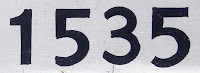NGC 1535 is a nebula in the constellation Eridanus.

## Wednesday, August 24, 2011

### 153

153 = 32 x 17.

153 is the 17th triangular number. It is also a hexagonal number.

153 = 1! + 2! + 3! + 4! + 5!

153 is palindromic in base 2 (binary): 10011001. It is 2121 in base 4 and 99 in base 16.

153 is the smallest number greater than 1 such that the sum of the cubes of its digits equals itself: 153 = 13 + 53 + 33.

153 has a representation as a sum of two squares: 153 = 32 + 122.In the Bible, Simon Peter is said to have caught 153 fish (John 21:11).

## Tuesday, August 23, 2011

### 1414

1414 = 2 x 7 x 101.

1414 is a divisor of 9515 - 1.

1414 is the smallest number whose square contains three consecutive nines: 14142 = 1,999,396.

14143 = 2,827,145,944 = (-28 + 27 + 1459 - 44)3.

1414 is 4060 in base 7 and 644 in base 15.Studio 1414 is the second largest sound stage in Minnesota.

## Monday, August 22, 2011

### 1239

1239 = 3 x 7 x 59.

1239 is a value of n for which n8, n9, n10, and n11 have the same digit sum (135).

1239 is the average of three successive primes (1231, 1237, 1249).

The sum of the squares of the factors of 1239 is a prime number: 32 + 72 + 592 = 3539, a prime.1239 is the composite number displayed at the start of the music video "Prime Composition" by Daniel Jones.

## Friday, August 19, 2011

### 675

675 = 33 x 52.

675 is the smallest order for which there are 17 groups.

6752 = 455,625 = (45 + 5 + 625)2.

675 is 300 in base 15. It is 10200 in base 5 and 120 in base 25.Triumph manufactures the Daytona 675 sports motorcycle.

## Thursday, August 18, 2011

### 378

378 = 2 x 33 x 7.

378 is the 27th triangular number. It is also a hexagonal number and the smallest Smith triangular number.

378 is the smallest three-digit number in which the sum of the digits is equal to the sum of the prime factors: 3 + 7 + 8 = 2 + 3 + 3 + 3 + 7 = 18.

378 is a cake number.378 Holmia is an asteroid discovered in 1893.

## Wednesday, August 17, 2011

### 1184

1184 = 25 x 37.

1184 has a representation as a sum of two squares: 1184 = 202 + 282.

1184 is one of a pair of amicable numbers. This is the first pair greater than 1000: 1184 and 1210.

1184 is 1121212 in base 3, 14214 in base 5, 5252 in base 6, 3311 in base 7, 1555 in base 9, and 828 in base 12. It is 987 in base 11.NGC 1184 is a galaxy in the constellation Cepheus.

## Tuesday, August 16, 2011

### 964

964 = 22 x 241.

964 is the number of 3 x 3 sliding puzzle positions that require exactly 12 moves to solve starting with the hole in the center.

964 has a representation as a sum of two squares: 964 = 82 + 302.

964 has the representation 964 = 210 - 60.

964 is a divisor of 156 - 1.

964 is 444 in base 15.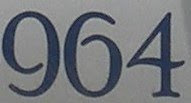The Porsche 964 was the company's internal name for a version of the Porsche 911 manufactured between 1989 and 1994.

## Monday, August 15, 2011

### 558

558 = 2 x 32 x 31.

558 is a divisor of 253 - 1. It is also a divisor of the sum of the largest prime factors of the first 558 positive integers.

558 in base 3 and base 4 starts off with the same three digits: 202200 and 20232.

The sum of the digits of 558 is six times the number digits: 5 + 5 + 8 = 18 = 6 x 3. 558 and 5582 (= 311,364) have the same digit sum.

558 is the sum of no fewer than 17 fourth powers.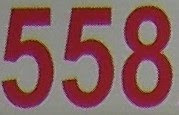558 is in the title of an episode of Star Trek: Deep Space Nine: "The Siege of AR-558".

## Friday, August 12, 2011

### 240

240 = 24 x 3 x 5.

240 is 11110000 in base 2 (binary).

240 is the number of equivalent hyperspheres in 8 dimensions that can touch an equivalent hypersphere without any intersections (kissing number).

240 is the smallest number with 20 divisors.

240 is a divisor of 312 - 1.

240 is a number that cannot be written as a sum of three squares.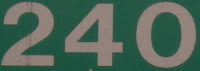240 is the number of distinct solutions of the Soma cube puzzle.

## Thursday, August 11, 2011

### 959

959 = 7 x 137.

959 is the first odd number that is not the sum of a prime and a power of two.

959 is a Carol number.

9592 = 919,681 = (-9 + 1 + 968 - 1)2.

959 is a divisor of 418 - 1.

959 is a number that cannot be written as a sum of three squares.The Porsche 959 was a sports car manufactured from 1986 t0 1989.

## Wednesday, August 10, 2011

### 890

890 = 2 x 5 x 89.

890 has two representations as a sum of two squares: 890 = 72 + 292 = 192 + 232.

890 is a divisor of 378 - 1.

890 is 1188 in base 9.

890 is the sum of four consecutive primes: 890 = 211 + 223 + 227 + 229.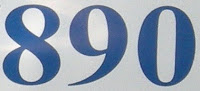WLS 890 AM is a radio station in Chicago.

Source: Wikipedia

## Tuesday, August 9, 2011

### 746

746 = 2 x 373.

746 has a representation as a sum of two squares: 746 = 112 + 252.

746 = 17 + 24 + 36.

746 x 8 x 92 x 531 = 291,548,736. Both sides of the equation use each of the digits from 1 to 9 once.

746 is the sum of two nonzero pentagonal pyramidal numbers: 746 = 550 + 196.A horsepower is a unit of power equal to about 746 watts.

## Monday, August 8, 2011

### 633

633 = 3 x 211.

633 is 1001111001 in base 2 (binary). It is 333 in base 14.

633 has the representation 633 = 36 - 96.

633 is a divisor of 555 - 1.

633 is the smallest number n whose fifth root has a decimal part that begins with the digits of n: 3.633128. . .

6333 = 253,636,137 = (2 - 5 + 3 + 636 + 1 + 3 - 7)3.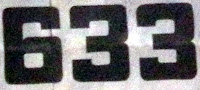633 Squadron was a 1964 British film that depicted the exploits of a fictional World War II fighter-bomber squadron.

## Friday, August 5, 2011

### 327

327 = 3 x 109. The digits of 327 are themselves all primes.

327 is largest number n such that n, 2n, and 3n contain every digit from 1 to 9 exactly once: 327, 654, 981. In other words, the sum 327 + 654 = 981 contains each of the digits from 1 to 9 once.

327 is the number of 5s among the digits of all four-digit primes.

327 is a divisor of 764 - 1.

327 is a number that cannot be written as a sum of three squares. It is the sum of four but no fewer squares.The BMW 327 was a touring car produced between 1937 and 1941.

## Thursday, August 4, 2011

### 440

440 = 23 x 5 x 11.

440 is the sum of the first 17 primes (2 to 59).

440 is divisible by the sum of its prime factors: 2 + 2 + 2 + 5 + 11 = 22.

440 is the number of permutations of 12 items that fix nine elements.

440 and 441 are two consecutive numbers, each divisible by a square.440 is the number of yards in the quarter-mile race in track-and-field competitions.

## Wednesday, August 3, 2011

### 606

606 = 2 x 3 x 101.

606 is both a 11-gonal (hendecagonal) number and a centered 11-gonal (hendecagonal) number.

606 is 4411 in base 5.

606 is the sum of six consecutive primes: 606 = 89 + 97 + 101 + 103 + 107 + 109.606 is the telephone area code the covers the eastern part of the state of Kentucky.

## Tuesday, August 2, 2011

### 303

303 = 3 x 101.

303 is the number of bipartite graphs on eight nodes (vertices).

3033 = 27,818,127, which is a concatenation of the cubes 1, 8, and 27.

303 is a divisor of 1012 - 1.

303 is a number that cannot be written as a sum of three squares.In the Gregorian calendar, 303 is the number of years that are not leap years in a period of 400 years.

## Monday, August 1, 2011

### 521

521 is a prime number.

521 has a representation as a sum of two squares: 521 = 112 + 202.

521 is the 13th Lucas number.

521 and 523 for a twin prime pair.

521 is the hypotenuse of a primitive Pythagorean triple: 5212 = 2792 + 4402.

521 has the representation 521 = 29 + 9.

521 is the exponent of the Mersenne prime 2521 - 1.

521 is the smallest prime whose reversal is a cube: 125 = 53.Galaxy cluster Abell 521 is located about 2.9 billion light-years from Earth.